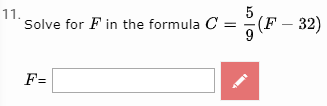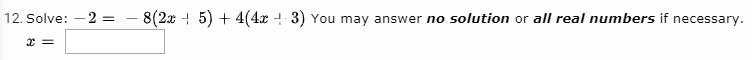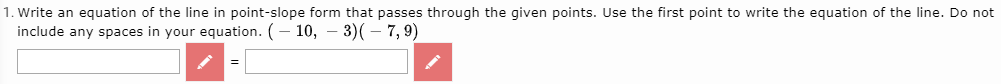## Allowable symbols in math expressions

Follow

I thought I posted this a few days ago, but now I don't see it. Apologies in advance if it is a dual post.

My question pertains to math exact and/or equivalent fill-in the blank questions. I know that symbols like =, <, and > aren't allowed. For example, if I put the correct answer is x+5=9, and the student types x+5=jslkawelrkljlads, Buzz will count that correct. Buzz compares the student answer to the correct answer, but when it sees the = it stops looking. Since the part before the = (x+5) matches the correct answer it is marked as correct.

I recently discovered that a semicolon also triggers this behavior.

Can you please provide a complete list of symbols that are unrecognized by Buzz in math expressions? That would save a lot of time and frustration of leaning by trial and error.

Thanks!

Sort by

Hello Bruce!

Unfortunately we don't have a list to provide of symbols that are unrecognized by Buzz in math expressions. But if there are examples of symbols that are not being recognized, we can investigate those cases to improve the system to account for more symbols.

0

Thanks Brian. Let me give you and example and maybe someone there can come up with a better way.

Let's say the question is to solve x^2+5x+6=0.

The correct solutions would be -2 and -3.

Ideally, we could use multiple fill-in the blanks and have the answers like this:

a. -3

b. -2

But if the student typed -2 in the first blank and -3 in the second it would be marked wrong. So next, this comes to mind:

a. -3

-2

b. -2

-3

Now Buzz will accept either answer for either blank, but now a student could put -2 in both blanks and get full credit. I've see different teachers use different ways around this issue. They basically come down to telling the students that they must put the answers in ascending order (which is awkward, not the best math practice, leads to frustration for students if they forget and type the correct answers in the wrong order). Or they use one answer blank with something to separate the answer such as:

a. -3;-2

-2;-3

This method allows the students to type the answers in either order. Because of the semicolon we can't use Match Exact so we have to set the question type as Normal. The problem is once again an issue of frustration for students who accidentally use a comma or include a space to type their correct answer since Normal looks for the exact same string of characters.

Any suggestions would be greatly appreciated!

0

Bruce - We are in the same boat as you. We would like to utilize the Math expression fill-in the blank type of questions for our math courses but it is becoming cumbersome to determine all of the different variations to that a student could potential make a mistake if we use the Normal questions type.  But the math expression types do not allow for math symbols such as = or < >.  This either makes it so we have to use the essay question or frustrated students who put in the correct answer but make a typo as you describe above.

Have you figured anything out that is working for you?  I am interested to see what workarounds are out there or if Agilix has improved the math expression type.

Thanks in advance for any suggestions.

0

Hi Tami,

Unfortunately there isn't a fix for this. I have been told that we could use the question type RegEx. I haven't had time to teach myself how regular expressions work though.

Later today I'll try to post a few work-arounds we use. If you have any specific questions reply with them here and I'll let you know how we deal with it.

0

So one thing we do for things like slope-intercept form or this literal equation below is to write variable = ______. That way they are only writing an expression instead of an equation or inequality.You can also have students type text in a math type question. I think it just reads the letters as variables, but it seems to work. Note this also works for having students put units on numbers.We also use 2 answer blanks with the equal sign so they are only entering expressions.0

Bruce,

Thank you for these examples.  This is very helpful.

Tami

0

No problem. Let me know if you need anything specific.

0